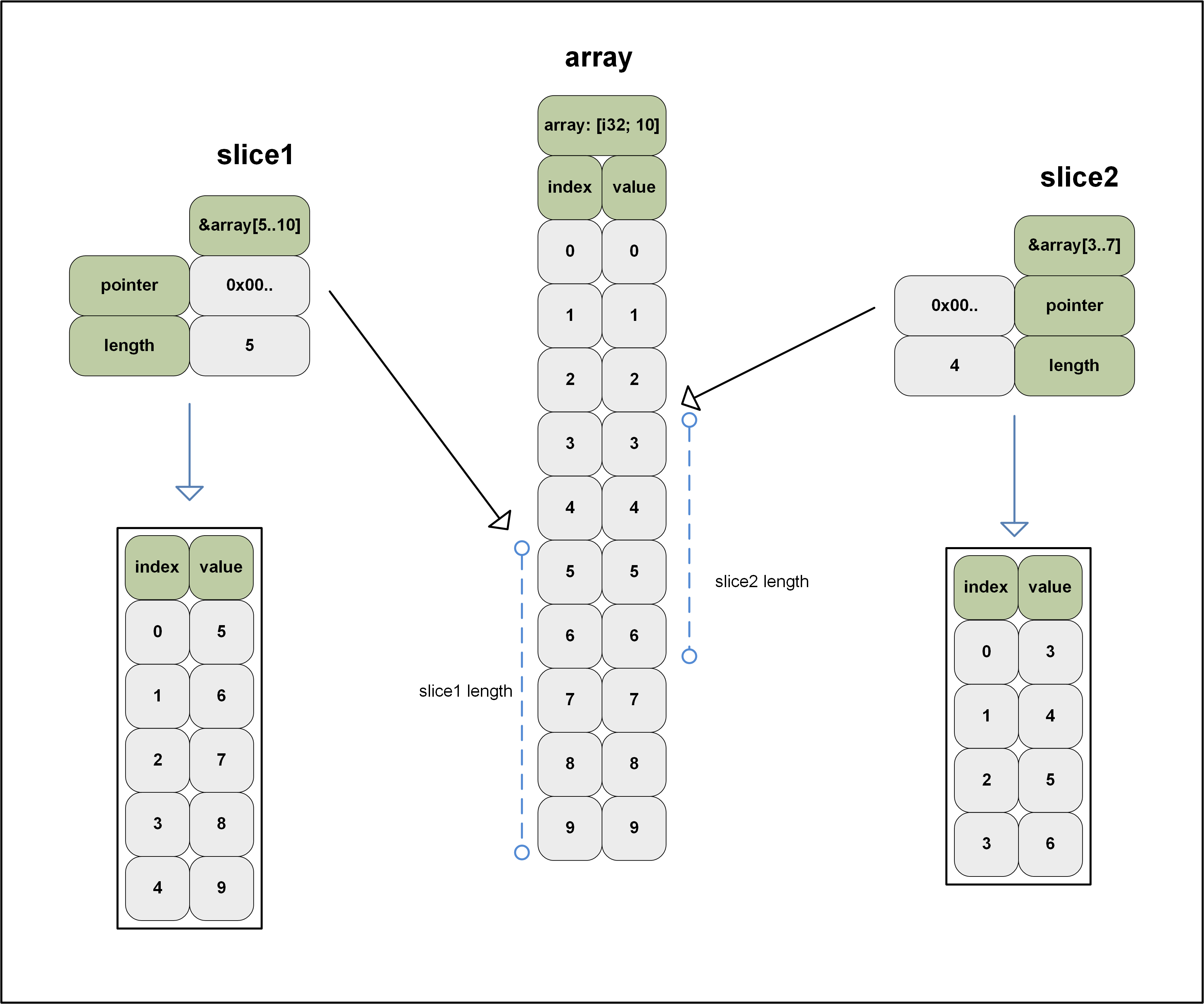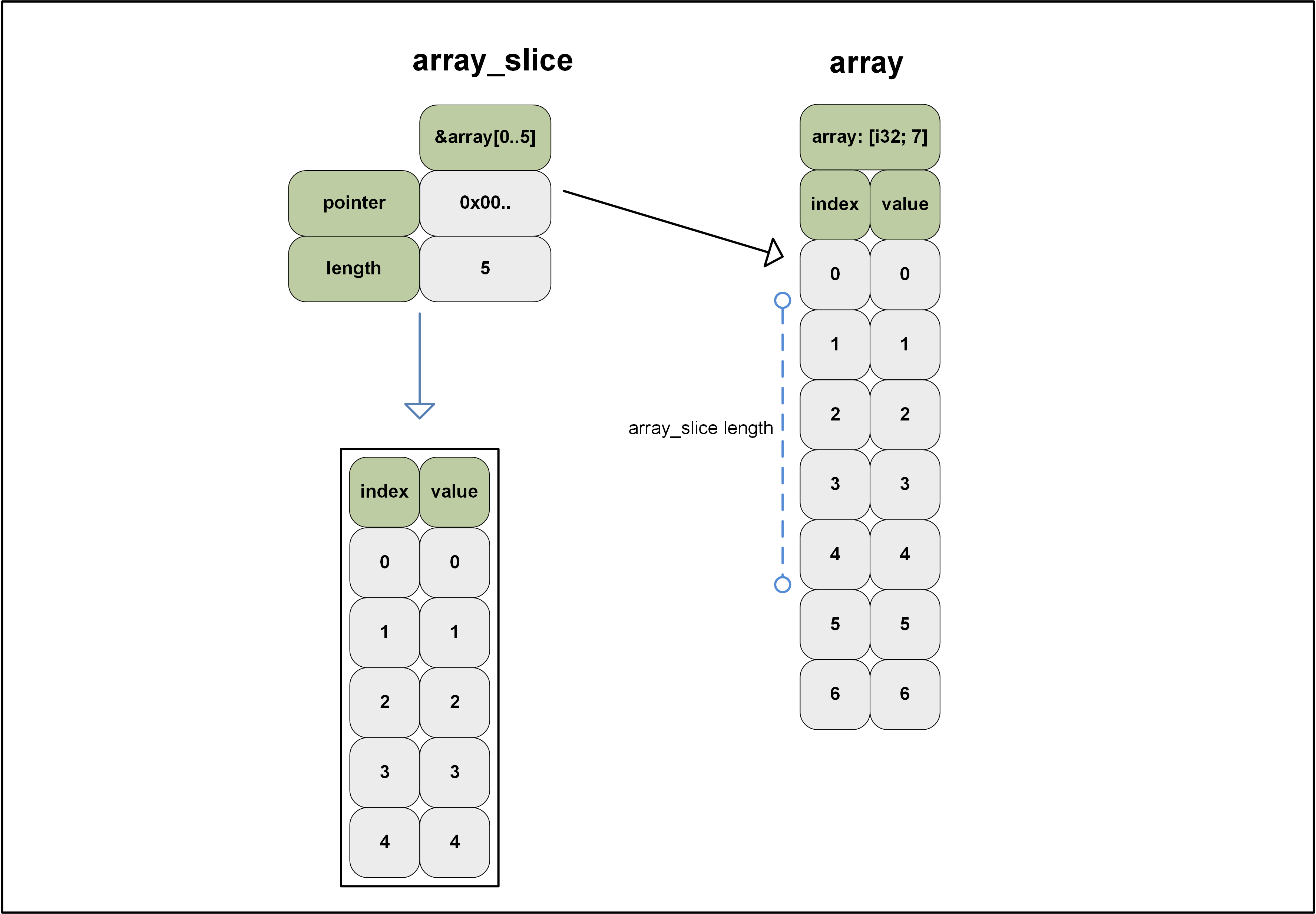In Rust, the `slice` is a `primitive type` as well as a `sequence type`. I found slices very confusing at first. In the Rust book, slices are defined as ‘a dynamically-sized view into a contiguous sequence’.

But somewhere in the rust-lang Github repo, the slice is defined as follows:

```Slices are a view into a block of memory represented as a pointer and a length.
```

I found this more helpful. It tells us the slice is a `fat pointer`. So basically, when you have a slice of an array, the slice contains the following:

• a pointer to the address of the element in the array that the slice starts with
• a value that describes the length of the slice

In Rust, the slice can be a view into a backing array as well as a view into other sequences, like vectors or Strings. In case the slice is a view into a `String`, it is called a `string slice`/`string literals` and it is usually seen in its borrowed form `&str`.

The following shows an example array and 2 slices from that array:On the left and on the right, we see slices that offer a view into the array that is shown in the middle. The array and the slices were defined as follows:

``````let array: [i32; 10] = [0, 1, 2, 3, 4, 5, 6, 7, 8, 9];
let slice1 = &array[5..10];
let slice2 = &array[3..7];
``````

In `slice1`, the pointer of the slice is pointing to array index 5. We can also see `slice1` has a length of 5. This means the slice will contain 5 elements of the array. A little below the slice, we can see the index and the values associated with `slice1`. The index of the slice itself runs from 0 to 4 and the values are those we can see in the backing array.

On the righthand side, we see `slice2`. That slice’s pointer is pointing to element 3 and the slice has a length of 4.

### Common slice operations

We start of defining and array and look at a few ways to take a slice:

``````let array: [i32; 7] = [0, 1, 2, 3, 4, 5, 6];

let slice = &array[..]; // [ 0, 1, 2, 3, 4, 5, 6 ]
let slice = &array[0..3]; // [ 0, 1, 2 ]
let slice = &array[..3]; // [ 0, 1, 2 ]
let slice = &array[2..4]; // [ 2, 3 ]
let slice = &array[2..]; // [ 2, 3, 4, 5, 6 ]
``````

The above shows an immutable array and a few ways in which we could create a slice out of it. The comment after the slice definition displays the `dbg!(slice);` output.

Let’s proceed and create a mutable slice so we can also change the value later on. We can do that as follows:

``````let mut array: [i32; 7] = [0, 1, 2, 3, 4, 5, 6];
let array_slice = &mut array[0..5]; // [ 0, 1, 2, 3, 4 ]
``````Checking the length of the slice and iterating the index / value:

``````slice.len(); // 5

for (index, item) in slice.iter().enumerate() {
println!("index: {:?} element {:?}", index, item);
}
/*
index: 0 element 0
index: 1 element 1
index: 2 element 2
index: 3 element 3
index: 4 element 4
*/
``````

Retrieving a value from the slice:

``````slice; // 1
``````

The slice length is not (always) known at compile time. The compiler will not save you in case you access an index value that is out of bounds:

``````slice;
``````

Running the above for our slice will result in the following:

```thread 'main' panicked at 'index out of bounds: the len is 5 but the index is 100'
```

To safely access values from a slice, we can use `get`:

``````slice.get(2); // Some(2)
slice.get(100); // None
``````

Finding values in a slice:

``````slice.iter().position(|v| v == &120); // None
slice.iter().position(|v| v == &4); // Some(4)
``````

Changing the value of an element in the slice:

``````slice = 100;
dbg!(slice); // [100, 1, 2, 3, 4]
dbg!(array); // [100, 1, 2, 3, 4, 5, 6]
``````

After we changed the value of an element, we can see that change reflected in the slice as well as in the underlying array that owns the values references by the slice.

### Slices out of different types

Slices can be taken out of arrays, vectors and Strings:

``````let array: [i32; 4] = [0, 1, 2, 3];
let array_slice = &array[..2]; // [0, 1]
let vector = vec![1, 2, 3, 4];
let vector_slice = &vector[0..2]; // [1, 2]
let string = String::from("string slice");
let string_slice = &string[0..6]; // "string"
println!("{:?} {:?} {:?}", array_slice, vector_slice, string_slice);
// [0, 1] [1, 2] "string"
``````

The previously defined array and vector contain the `i32` type. For this reason, we can create a function that works on both the `vector_slice` as well as the `array_slice`:

``````fn return_second(n: &[i32]) {
println!("{}", n);
}
return_second(array_slice); // 1
return_second(vector_slice); // 2
``````

The string slice is a `&str`, so we would not be able to pass it into the `return_second` function. In fact, the string slice is a bit ‘special’. All strings in Rust are UTF-8 and therefore, characters can differ in size. The `iter()` does not work on string slices, instead, we need to turn to `chars()`. And to take the nth character from a slice, we will need to turn to `nth()`:

``````let string = String::from("Rust is 😍");
let string_slice = &string[..];

fn return_second_char(n: &str) {
println!("{:?}", n.chars().nth(1));
}

return_second_char(string_slice); // Some('u')

for c in string_slice.chars() {
println!("{}", c)
}
/*
R
u
s
t

i
s

😍
*/
for (i, c) in string_slice.chars().enumerate() {
println!("{} {}", i, c)
}
/*
0 R
1 u
2 s
3 t
4
5 i
6 s
7
8 😍
*/
``````

### Fat and thin pointers

In closing, some additional words on fat and thin pointers. While I was reading up on slices, I got completely hung up on them for several reasons. I did not really understand the difference between a reference to an array and a slice and several other factors were making the slice a bit of a grey area.

A colleague, (Nate Newton), wrote some code for me that helped me understand it a bit better. Just being able to ‘see’ sometimes helps me better understand things. And what he showed me was something along these lines:

``````use std::mem;
let array: [i32; 500] = [0; 500];
let slice = &array[..];
let array_pointer = &array;
let slice_pointer = &slice;
let start_of_array_slice = &array;
println!("--------------------------------------------");
println!("slice occupies {} bytes", mem::size_of_val(&slice));
println!(
"array_pointer occupies {} bytes",
mem::size_of_val(&array_pointer)
);
println!("array occupies {} bytes", mem::size_of_val(&array));
println!("--------------------------------------------");
``````

The above outputs the following:

```--------------------------------------------